AP State Syllabus AP Board 9th Class Maths Solutions Chapter 1 Real Numbers Ex 1.3 Textbook Questions and Answers.

## AP State Syllabus 9th Class Maths Solutions 1st Lesson Real Numbers Exercise 1.4

Question 1.
Simple the following expressions.
i) (5 + √7) (2 + √5)
Solution:
(5 + √7) (2 + √5)
= 10 + 5√5 + 2√7 + √35

ii) (5 + √5) (5 – √5)
Solution:
(5 + √5) (5 – √5)
= 52 + (√5)2
= 25 – 5 = 20(iii) (√3 + √7)2
Solution:
(√3 + √7)2
= (√3)2 + (√7)2 + 2(√3)(√7)
= 3 + 7 + 2√21
= 10 + 2√21

iv) (√11 – √7) (√11 + √7)
= (√11)2 – (√7)2
= 11 – 7 = 4

Question 2.
Classify the following numbers as rational or irrational.
i) 5 – √3
ii) √3 + √2
iii) (√2 – 2)2
iv) $$\frac{2 \sqrt{7}}{7 \sqrt{7}}$$
v) 2π
vii) (2 +√2) (2 – √2)
Solution:
i) 5 – √3 – irrational
ii) √3 + √2 – irrational
iii) (√2 – 2)2 – irrational
iv) $$\frac{2 \sqrt{7}}{7 \sqrt{7}}$$ – rational
v) 2π – Transcendental number. (not irrational)
vi) $$\frac{1}{\sqrt{3}}$$– irrational
vii) (2 +√2) (2 – √2) – rationalQuestion 3.
In the following equations, find whether variables x, y, z etc., represents rational or irrational numbers.
i) x2 = 7
ii) y2 = 16
iii) z2 = 0.02
iv) u2 = $$\frac{17}{4}$$
v) w2 = 27
vi) t4 = 256
Solution:
i) x2 = 7
⇒ x = √7 is an irrational number.
ii) y2 = 16 ⇒ y = 4 is a rational number.
iii) z2 = 0.02 ⇒ z = $$\sqrt{0.02}$$ is an irrational number.
iv) u2 = $$\frac{17}{4}$$ ⇒ x = $$\frac{\sqrt{17}}{2}$$ is an irrational number.
v) w2 = 27 ⇒ w = $$3 \sqrt{3}$$ an irrational number.
vi) t4 = 256 ⇒ t2 = $$\sqrt{256}$$ = 16
⇒ t = $$\sqrt{16}$$ = 4 is a rational number

Qeustion 4.
The ratio of circumference to the diameter of a circle c/d is represented by π. But we say that π is an irrational number. Why?

Question 5.
Rationalise the denominators of the following.
i) $$\frac{1}{3+\sqrt{2}}$$
Solution: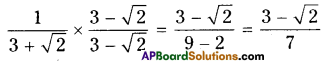ii) $$\frac{1}{\sqrt{7}-\sqrt{6}}$$
Solution: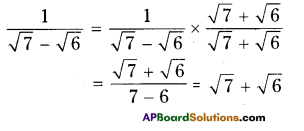iii) $$\frac{1}{\sqrt{7}}$$
Solution: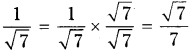iv) $$\frac{\sqrt{6}}{\sqrt{3}-\sqrt{2}}$$
Solution: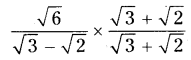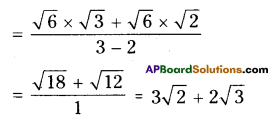Question 6.
Simplify each of the following by rationalising the denominator.
i) $$\frac{6-4 \sqrt{2}}{6+4 \sqrt{2}}$$
Solution: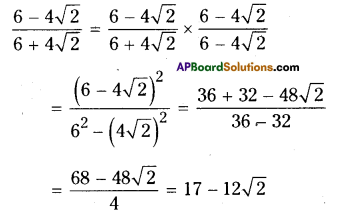ii) $$\frac{\sqrt{7}-\sqrt{5}}{\sqrt{7}+\sqrt{5}}$$
Solution:iii) $$\frac{1}{3 \sqrt{2}-2 \sqrt{3}}$$
Solution: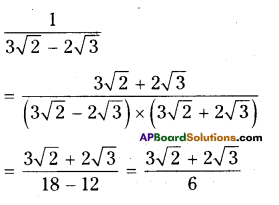iv) $$\frac{3 \sqrt{5}-\sqrt{7}}{3 \sqrt{3}+\sqrt{2}}$$
Solution: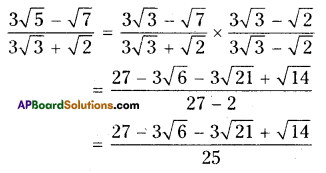Question 7.
Find the value of $$\frac{\sqrt{10}-\sqrt{5}}{2 \sqrt{2}}$$ upto three decimal places. (take $$\sqrt{2}$$ = 1.414, $$\sqrt{3}$$ = 1.732 and $$\sqrt{5}$$ = 2.236).
Solution: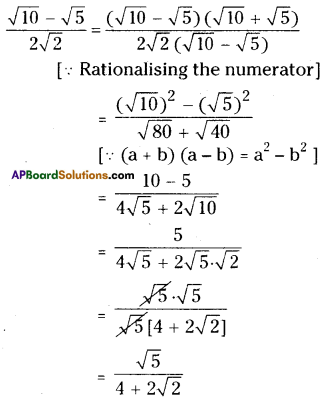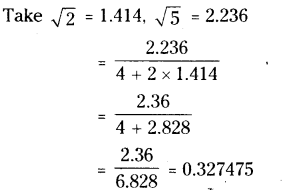Question 8.
Find
i) 641/6
Solution:
= (26)1/6
= 6

ii) 321/5
Solution:
321/5
= (25)1/5
= 2

iii) 6251/4
6251/5
= (54)1/4
= 5

iv) 163/2
Solution:
163/2
= (42)3/2

v) 2432/5
Solution:
2432/5
= (35)2/5

vi) (46656)-1/6
Solution: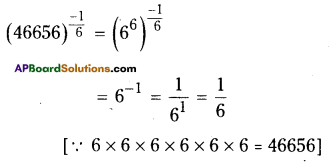Question 9.
Simplify $$\sqrt{81}-8 \sqrt{343}+15 \sqrt{32}+\sqrt{225}$$
Solution: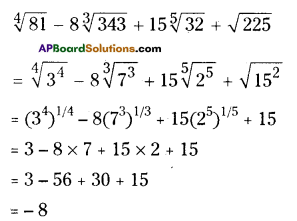Question 10.
If ‘a’ and ‘b’ are rational numbers, find the values of a and b in each of the following equations.
i) $$\frac{\sqrt{3}+\sqrt{2}}{\sqrt{3}-\sqrt{2}}=\mathbf{a}+\mathbf{b} \sqrt{6}$$
Solution:
Given that $$\frac{\sqrt{3}+\sqrt{2}}{\sqrt{3}-\sqrt{2}}=\mathbf{a}+\mathbf{b} \sqrt{6}$$
Rationalising the denominator we get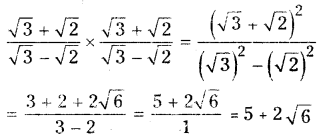Comparing 5 + 2√6 with a + b√6
We have a = 5 and b = 2ii) $$\frac{\sqrt{5}+\sqrt{3}}{2 \sqrt{5}-3 \sqrt{3}}=a-b \sqrt{15}$$
Solution:
Given that $$\frac{\sqrt{5}+\sqrt{3}}{2 \sqrt{5}-3 \sqrt{3}}=a-b \sqrt{15}$$
Rationalising the denominator we get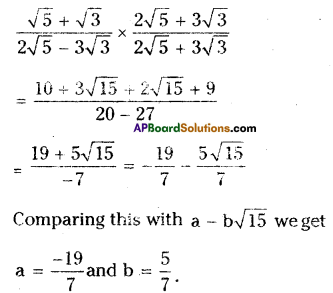AP Board 9th Class Maths Solutions